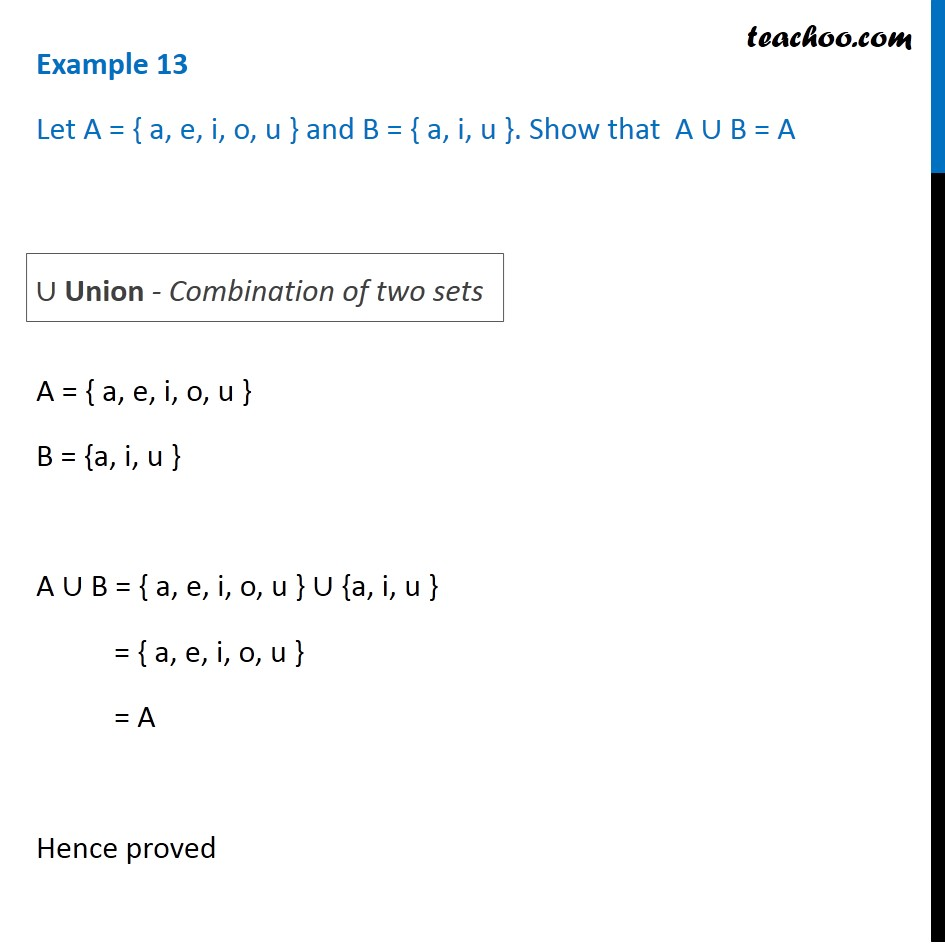Examples

Chapter 1 Class 11 Sets
Serial order wiseLearn in your speed, with individual attention - Teachoo Maths 1-on-1 Class

### Transcript

Example 13 Let A = { a, e, i, o, u } and B = { a, i, u }. Show that A ∪ B = A A = { a, e, i, o, u } B = {a, i, u } A ∪ B = { a, e, i, o, u } ∪ {a, i, u } = { a, e, i, o, u } = A Hence proved ∪ Union - Combination of two sets